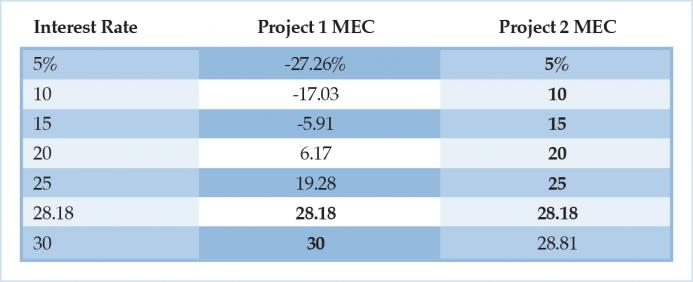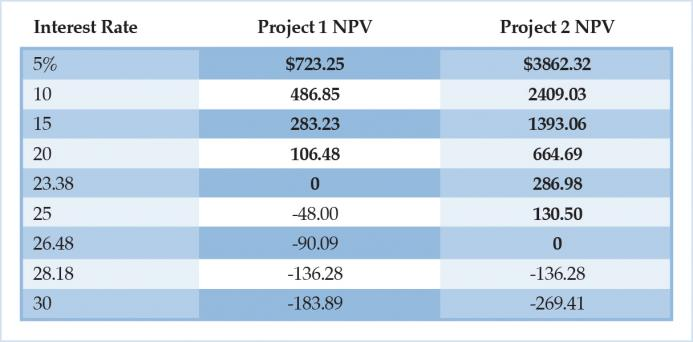The Journal of Libertarian Studies

Home | Mises Library | The Marginal Efficiency of Capital: A Comment

The Marginal Efficiency of Capital: A Comment

•0 Views
01/30/2015

Volume 17, No. 4 (Winter 2014)

KEYWORDS: John Maynard Keynes, marginal efficiency of capital, net present value, interest rates, central bankingJEL CLASSIFICATION: E12, E22, E52,

In his recent article, Edward W. Fuller (2013) compared the Keynesian Marginal Efficiency of Capital approach with the Austrian Net Present Value approach. While his article has some important insights regarding the different treatments of investment projects in these two approaches, the result that the two approaches result in different rankings will only hold if factor prices are held constant. But, as the paper states, such an assumption is generally not true.

To briefly summarize Fuller’s main point: the net present value criterion demonstrates that there is a “switching” from one type of investment project to another as interest rates change. In particular, as interest rates rise, shorter projects will be preferred, while longer projects are preferred when interest rates are lower. In the marginal efficiency of capital approach, there is no such switching. Rather, there is an invariant list of projects with each listed by its rate of return (defined as that interest rate which sets the net present value equal to zero), and the going interest rate acts as a “hurdle” rate, determining how far down the list investors will go when funding projects.

All of this is true, if we hold the cost of starting the projects (and therefore the rate of return) constant. However, if we include the insight that “[c]ompetition between investors creates a tendency for the net present value of an investment project to equal zero” (Fuller, 2013, p. 381), then these results fail to hold. To show this, I will slightly modify Fuller’s examples.

Suppose that we have two projects that would utilize the same resources, so entrepreneurs with these two projects in mind are bidding against one another. The first project (“Project 1”) pays \$1,000 of positive cash flow in each of the next three years (equivalent to Fuller’s “wooden bridge”) The second project (“Project 2”) pays \$1,000 for each of 8 years, starting 3 years from now (equivalent to Fuller’s “steel bridge”). Fuller assumes that the first project will cost \$2,000 to start, while the second costs \$5,000. That is where the problem lies: if competitive bidding occurs, then the starting cost is not fixed. It will depend on the interest rate, and the Net Present Value (NPV) of the project with greater present value will be zero, while the less valuable project’s NPV will be negative. In short: while it is true that, “other things equal”, as the interest rate changes, the NPV will change as described by Fuller, Fuller has argued that when the interest rate changes, the startup cost of the project will change as well—and will change to keep the NPV at zero for any projects that get funded. To reexamine Fuller’s point, we calculate the Present Values (not the Net Present Values), under the assumption that the two projects are competing ways of using the same set of resources.

Table 1. Present Values

As long as the interest rate is below 28.18 percent, the longer project has a higher present value, so entrepreneurs pursuing Project 2 will get control of the resources and pursue that project. If interest rates are above 28.18 percent, then the shorter project will have a greater present value, so entrepreneurs that pursue Project 1 will win control of the resources and pursue that project.On the whole, the story here is very similar to Fuller’s, simply because Fuller’s NPV was really just present value, but subtracting an arbitrary constant that he treated as the startup cost. However, the story changes if we allow for the startup cost to change and then look at the marginal efficiency of capital (MEC) criterion. To calculate the MEC, first I assume an interest rate. Then, I calculate the present value of the two projects. Then, I assume that the project’s startup cost is equal to the greater of the two present values. Then, I calculate the interest rate that would be required to make the Net Present Value of each project zero.

Table 2. Marginal Efficiencies of Capital

Once we correct for the changing cost of startup, the net present value and marginal efficiency criteria will give the same ordering—Project 2 is preferred if the interest rate is less than 28.18 percent, Project 1 is preferred if the interest rate is more than 28.18 percent. The reason is that the net present value of the “winning” project is zero, so the MEC of the winning project is equal to the going interest rate. The “losing” project has a negative NPV. To increase the NPV to zero, the MEC must be below the going interest rate used to calculate the original NPV.But, what if we allow that the startup costs may be fixed? Does that suggest the rank ordering will be different for the two projects? Yes and no. Fuller has already laid out the reasons for a “yes” answer, so let me present the reasons for the “no.” If we apply the net present value criterion correctly, the decision we are making is not which of two (or more) projects to select—it is whether we should pursue a particular project at all. If the NPV is equal to or greater than zero, then investing in the project is wealth-enhancing. If the NPV is less than zero, then investing in the project is wealth-diminishing. In the following table, I assume that the startup cost is always \$2,000, and bold those projects that should be undertaken. Then, I calculate the MEC for each project, assuming a \$2,000 startup cost.

Table 3. Net Present Values (fixed startup cost of \$2,000)

Under these assumptions, if the interest rate is less than 23.38 percent, then both Project 1 and Project 2 are undertaken according to the NPV criterion. If the interest rate is less than 26.48 percent, but greater than 23.38 percent, then Project 2 is undertaken, but Project 1 is not. If the interest rate is greater than 26.48 percent, then neither project is undertaken. By definition, the MEC of Project 1 is the interest rate that makes the NPV zero—so 23.38 percent. By definition, the MEC of Project 2 is 26.48 percent. So, using the marginal efficiencies of capital and comparing to a hurdle rate gives the same result as looking for a net present value greater than zero.All of that said, Fuller raises an interesting point: Austrian theory is primarily about which investment projects get chosen, while Keynesian theory is driven by the question of how many projects get chosen. The goal of this comment is to add some clarification for two underlying reasons for those differences. The first reason is that Keynesian theory assumes idle resources. The second reason flows from that assumption: in Keynesian theory, prices of starting investment projects do not fully reflect expected, discounted present values of those projects—instead startup costs are “sticky.” Thus, Austrians, focusing on unsustainable malinvestments, see credit expansion as destructive while, for Keynesians, “[t]he conception of the interest rate as a hurdle rate naturally leads to a monetary policy of manipulating the interest rate.” (Fuller, 2013, p. 394)

References

Fuller, Edward W. “The Marginal Efficiency of Capital.” Quarterly Journal of Austrian Economics 16, no. 4 (Winter 2013): 379–400.